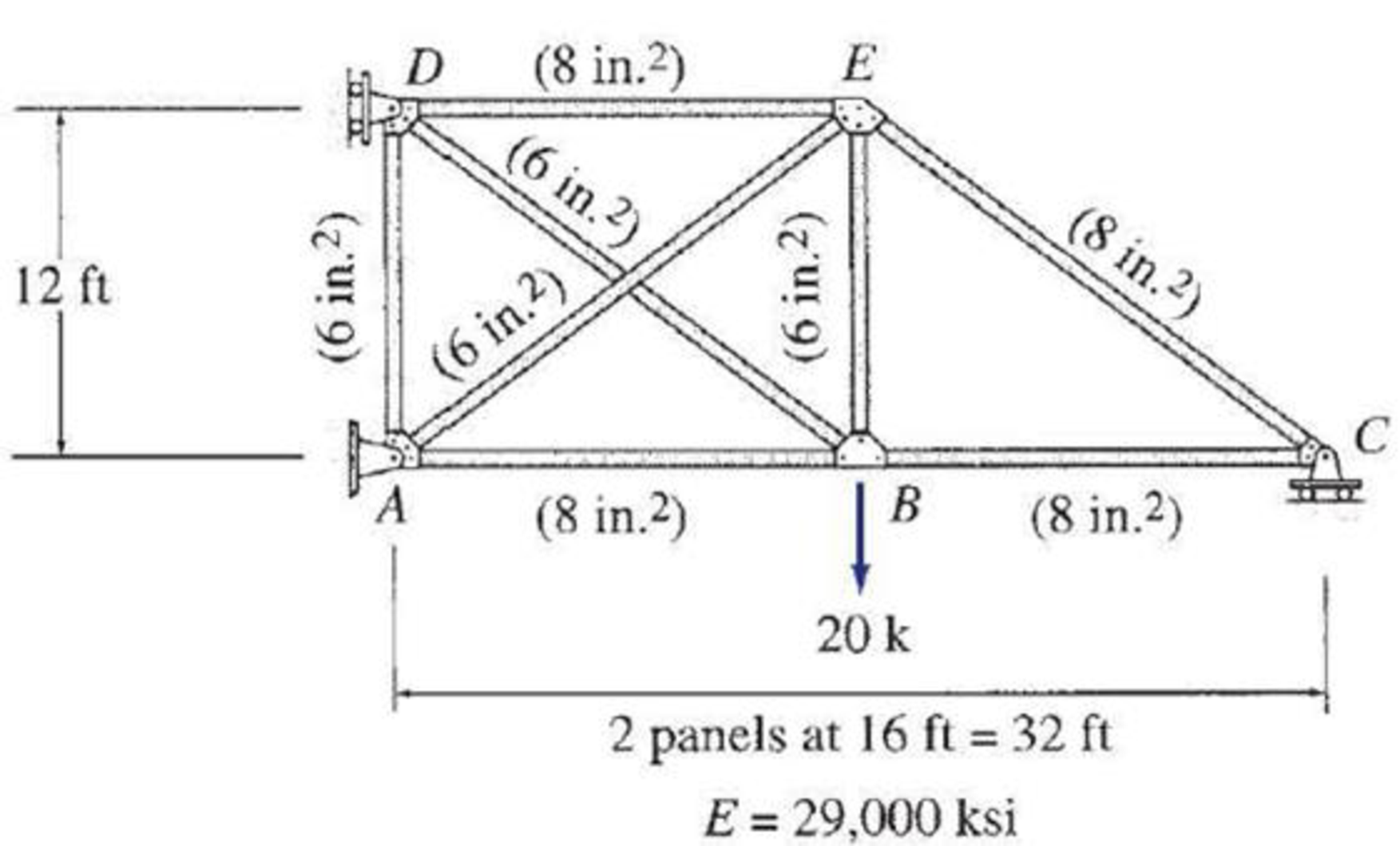# 13.46 and 13.47 Determine the reactions and the force in each member of the trusses shown in Figs. P13.46 and P13.47 using the method of consistent deformations. FIG. P13.47

#### Solutions

Chapter
Section
Chapter 13, Problem 47P
Textbook Problem
691 views

## 13.46 and 13.47 Determine the reactions and the force in each member of the trusses shown in Figs. P13.46 and P13.47 using the method of consistent deformations.FIG. P13.47

To determine

Calculate the support reactions and the member forces of the truss using method of consistent deformation.

### Explanation of Solution

Given information:

The structure is given in the Figure.

Apply the sign conventions for calculating reactions, forces and moments using the three equations of equilibrium as shown below.

• For summation of forces along x-direction is equal to zero (Fx=0), consider the forces acting towards right side as positive (+) and the forces acting towards left side as negative ().
• For summation of forces along y-direction is equal to zero (Fy=0), consider the upward force as positive (+) and the downward force as negative ().
• For summation of moment about a point is equal to zero (Matapoint=0), consider the clockwise moment as negative and the counter clockwise moment as positive.

Method of joints:

The negative value of force in any member indicates compression (C) and the positive value of force in any member indicates tension (T).

Condition for zero force members:

1. 1. If only two non-collinear members are connected to a joint that has no external loads or reactions applied to it, then the force in both the members is zero.
2. 2. If three members, two of which are collinear are connected to a joint that has no external loads or reactions applied to it, then the force in non-collinear member is zero.

Calculation:

Sketch the free body diagram of the truss as shown in Figure 1.

Find the degree of indeterminacy of the structure using the equation:

i=m+r2j

Here, m is the number of members, r is the reaction of the truss, and j is the number of joints.

Substitute 8 for m, 4 for r, and 5 for j.

i=8+42(5)=1210=2

Therefore, the degree of indeterminacy of the truss is i=1.

Select the member BD and the vertical reaction at C as redundant.

Remove the redundant member BD and support at C.

Sketch the free body diagram of the truss without member BD and support at C as shown in Figure 2.

Refer Figure 2,

Find the length of member AE (LAE)

LAE=162+122=20ft

Find the vertical reaction (AyO) at A.

Summation of forces along y-direction is equal to 0.

+ΣFy=0AyO20=0AyO=20k()

Find the horizontal reaction (AxO) at x.

Summation of moment at D is equal to 0.

+MD=020(16)+Ax0(12)=012AxO=320Ax0=26.67k

Summation of forces (FO) along x-direction is equal to 0.

+ΣFx=0

AxO+DxO=0DxO=26.67kDxO=26.67k()

Find the member forces using method of joints.

The zero force members are AD, BC, and CE.

Consider horizontal equilibrium at joint D.

+ΣFx=0

Dx+FODE=0

Substitute 26.67k for Dx.

(26.67)+FODE=0FODE=26.67k(T)

Consider vertical equilibrium at joint A.

+ΣFy=0

Substitute 20k for Ay and 0 for FOAD.

20+0+FOAE(1220)=00.6FOAE=20FOAE=33.33kFOAE=33.33k(C)

Consider vertical equilibrium at joint B.

+ΣFy=0

20+FOBE=0FOBE=20k(T)

Sketch the truss with member forces due to external loads as shown in Figure 3.

Remove all external forces and apply the unit load for redundant member BD.

Consider the forces in the member due to unit load as u.

Apply unit tensile load for member BD.

Sketch the free body diagram of the truss with unit load of member BD as shown in Figure 4.

Refer Figure 4.

The zero member forces are BC and CE.

Find the member force using proportion of length.

The force in member BD is 1 k tensile.

Find the member force AE.

uAE20=120uAE=1k(T)

Find the member force AB and DE.

uAB(or)uDE16=120uAB=uDE=0.8kuAB=uDE=0.8k(C)

Find the member force AB and DE.

Sketch the truss with member force due to unit load of member BD as shown in Figure 5.

Apply the unit load for redundant support at C.

Consider the forces in the member due to unit load for support as k.

Remove all external forces and apply unit upward load for at support C.

Sketch the free body diagram of the truss with unit load at support C as shown in Figure 6.

Refer Figure 6,

The zero force member is BE.

Find the member forces using method of joints.

Consider vertical equilibrium at point C.

+ΣFy=0

1+kCE(1220)0.6kCE=1kCE=1.67kkCE=1.67k(C)

Consider horizontal equilibrium at point C.

+ΣFx=0

kCBkCE(1620)=0

Substitute 1.67k for kCE.

kCB(1.67)(0.8)=0kCB=1.33(T)

Consider horizontal equilibrium at point B.

+ΣFx=0

kABkBC=0

Substitute 1.33k for kBC.

kAB1.33=0kAB=1.33k(T)

Consider vertical equilibrium at point E.

+ΣFy=0

kBEkAE(1220)kEC(1220)=0

Substitute 0 for kBE and 1.67k for kEC.

00.6kAE0.6(1.67)=0kAE=1.67k(T)

Consider horizontal equilibrium at point E.

+ΣFx=0

kED+kEC(1620)kAE(1620)=0kED=0

### Still sussing out bartleby?

Check out a sample textbook solution.

See a sample solution

#### The Solution to Your Study Problems

Bartleby provides explanations to thousands of textbook problems written by our experts, many with advanced degrees!

Get Started

Find more solutions based on key concepts
For Problem 19.33, calculate the probability distribution and plot the probability distribution curve.

Engineering Fundamentals: An Introduction to Engineering (MindTap Course List)

touchpad a. a type of 2-D bar code that stores information in both horizontal and vertical directions b. small,...

Enhanced Discovering Computers 2017 (Shelly Cashman Series) (MindTap Course List)

What are three safety rules to observe when using screwdrivers?

Precision Machining Technology (MindTap Course List)

What kind of data and information can be found using a packet .sniffer?

Principles of Information Security (MindTap Course List)

Provide an overview of the systems design phase.

Systems Analysis and Design (Shelly Cashman Series) (MindTap Course List)

If your motherboard supports ECC DDR3 memory, can you substitute non-ECC DDR3 memory?

A+ Guide to Hardware (Standalone Book) (MindTap Course List)

List two types of grinders used by welders.

Welding: Principles and Applications (MindTap Course List)